# How to Print Formula Syntax in ExcelIn this article, we will learn How to Print Formula Syntax in Excel.

Scenario :

Excel performs a function in a cell when using equals  to sign. For example, if you type = 2 + 2  in a cell it will result in 4. Excel lets you view formulas with the following given methods.

1. Via Show Formulas option
2. Using single quotation mark ( ‘ ) in front of the formula
3. Use Ctrl + ` (accent mark) to view all formulas

This usually happens when we have scattered data which cannot be edited unless we know where to edit. For example on a financial dataset, there are some prerequisites and some are derived results. Now when we need to edit some data, we double click any box and find that it has a formula, so start finding the prerequisites cells. So this method comes in handy to solve the problems. Let's understand how formulas in Excel are viewed with some examples to illustrate the option usage.

Example :

All of these might be confusing to understand. Let's understand how to use the formula using an example. Here we are given some values some are extracted using formula and some are not. So when required to change the data, we need to know where the formula exists.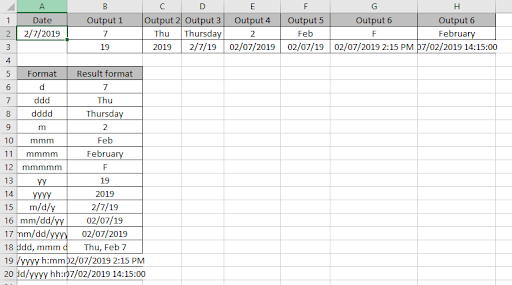Here we have some data where results have been extracted using Excel formulas. Go to tab Formulas > Show formulas under Formula Auditing.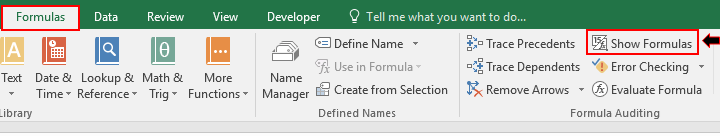Click the Show Formula option to view all formulas of the sheet or use the shortcut Ctrl + ` (tilde) from the keyboard.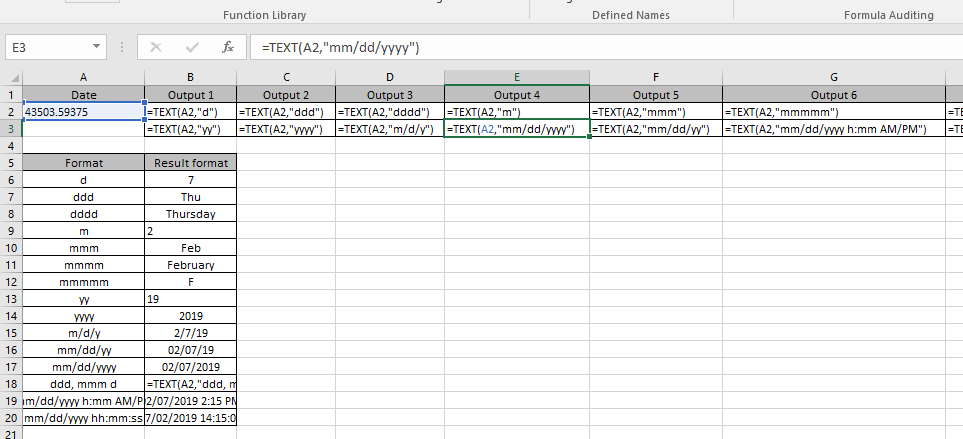This option lets you view all Excel Formulas used in the worksheet instead of the results.

There’s one more way to view excel formulas, not the result. If you wish to view the Formula of a particular cell. Then use the below-mentioned method.

Single Quote mark in front of the formula lets you show only formulas, not the result of the cell. Here I have used this method on an Excel formula.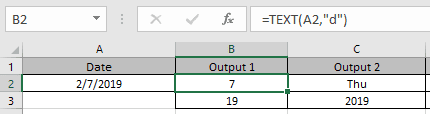Now I wish to view formulas on B2 & C3 cell here. Just apply one single quote mark in front of the formula.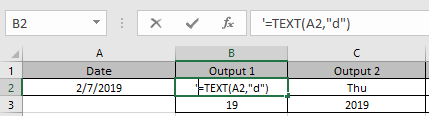Apply the same in C3 cell to view excel formula instead of results.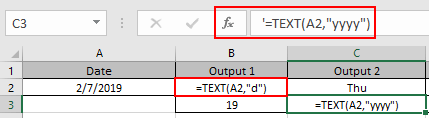As you can see in the above snapshot Excel formula not the result in the cell.

Here are some observational notes using the formula syntax:

Notes

1. Each Formula in excel starts with ( = ) sign.
2. Sometimes there exist a curly braces in the start of formula, which is an array formula and called using the Ctrl + Shift + Enter. Do not put curly braces manually.
3. Text values and characters are always given using quotes or using cell reference
4. Numerical values can be given directly or using the cell reference.
5. Array can be given using the array reference or using named ranges.

Hope this article about How to Print Formula Syntax in Excel is explanatory. Find more articles on calculating values and related Excel formulas here. If you liked our blogs, share it with your friends on Facebook. And also you can follow us on Twitter and Facebook. We would love to hear from you, do let us know how we can improve, complement or innovate our work and make it better for you. Write to us at info@exceltip.com.

Related Articles :

Evaluation of Excel Formula Step By Step : Excel provides functionality that helps us evaluate the formula. We can see how a formula is working by stepping through the formula.

How to use the Shortcut To Toggle Between Absolute and Relative References in Excel : F4 shortcut to convert absolute to relative reference and same shortcut use for vice versa in Excel.

How to use Shortcut Keys for Merge and Center in Excel : Use Alt and then follow h, m and c to Merge and centre cells in Excel.

How to Select Entire Column and Row Using Keyboard Shortcuts in Excel : Use Ctrl + Space to select whole column and  Shift + Space to select whole row using keyboard shortcut in Excel

Paste Special Shortcut in Mac and Windows : In windows, the keyboard shortcut for paste special is Ctrl + Alt + V. Whereas in Mac, use Ctrl + COMMAND + V key combination to open the paste special dialog in Excel.

How to Insert Row Shortcut in Excel : Use Ctrl + Shift + = to open the Insert dialog box where you can insert row, column or cells in Excel.

Popular Articles :